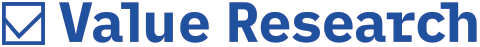# The alpha factor

##### Alpha tells you if a fund has produced returns justifying the risks assumedAlpha, as you may know, is the first letter of the Greek alphabet. But when analysts and investors use it in the context of modern portfolio theory, Greek is far from their mind. What they want to know is how well their fund has performed.

Alpha tells you whether a fund has produced returns justifying the risks it assumed. It does this by comparing its actual return to the one 'predicted' by the beta. Say, a fund can be expected to earn a return of 15 per cent in a year (based on its beta), but it actually fetches you 18 per cent. Then the alpha of the fund is three (18 - 15 = 3). In other words, alpha is a measure of selection risk (also known as residual risk) of a mutual fund in relation to the market. A positive alpha is the extra return awarded to the investor for assuming a risk, instead of accepting the market return.

Alpha may be seen as a measure of a fund manager's performance. It indicates what the fund has earned over and above (or under) what it was expected to earn. Thus, this is the value added (or subtracted) by the fund manager's investment decisions. A passive fund has an alpha of 0. That's why index funds always have or should have, if they track their benchmark index perfectly, an alpha of 0. An active fund's alpha is a measure of what the fund manager's activity has contributed to the fund's returns.

Alpha is the portfolio's risk-adjusted performance or the 'value added' by a manager. Mathematically, alpha is the incremental difference between a manager's actual results and his expected results, given the level of risk. A positive alpha indicates that a portfolio has produced returns above the expected level, and a negative alpha suggests the portfolio underperformed given the level of risk assumed. So, two fund managers may beat the same benchmark, but only one may outperform on a risk-adjusted basis. That one has got alpha.

Alpha is one of the five technical risk ratios used in Modern Portfolio Theory. The other four are beta, R-squared, standard deviation and the Sharpe Ratio. All these statistical measurements help investors determine the risk-reward profile of a mutual fund.